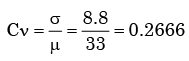Courses

# Probability And Statistics - MCQ Test 1

## 20 Questions MCQ Test Topicwise Question Bank for GATE Civil Engineering | Probability And Statistics - MCQ Test 1

Description
This mock test of Probability And Statistics - MCQ Test 1 for Civil Engineering (CE) helps you for every Civil Engineering (CE) entrance exam. This contains 20 Multiple Choice Questions for Civil Engineering (CE) Probability And Statistics - MCQ Test 1 (mcq) to study with solutions a complete question bank. The solved questions answers in this Probability And Statistics - MCQ Test 1 quiz give you a good mix of easy questions and tough questions. Civil Engineering (CE) students definitely take this Probability And Statistics - MCQ Test 1 exercise for a better result in the exam. You can find other Probability And Statistics - MCQ Test 1 extra questions, long questions & short questions for Civil Engineering (CE) on EduRev as well by searching above.
QUESTION: 1

### A fair dice is rolled twice. The probability that an odd number will follow an even number is

Solution:

Here the sample space S = 6

Therefore  P(odd number ) = 3/6 = 1/2

and  P (even number )  = 3/6 = 1/2

since events are independent,

therefore, P (odd / even )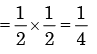QUESTION: 2

### Three companies X, Y and Z supply computers to a university. The percentage of computers supplied by them and the probability of those being defective are tabulated below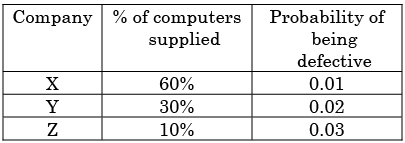Given that a computer is defective, the probability that it was supplied by Y is

Solution:

Probability of defective computer supplied by Y =
(Case when Y produces defective)/(All cases of producing defective product)
Case when Y produces defective = (0.3)(0.02) = 0.006
All cases of producing defective product= (0.6x0.01)+(0.3x0.02)
(0.1x0.03)= 0.006+0.006+0.003=0.015

Probability = 0.006/0.015=0.4

QUESTION: 3

### An examination consists of two papers, Paper 1 and Paper 2. The probability of failing in Paper 1 is 0.3 and that in Paper 2 is 0.2. Given that a student has failed in Paper 2, the probability of failing in Paper 1 is 0.6. The probability of a student failing in both the papers is

Solution:

Let A be  the event that ‘failed in paper 1’.

B be the event that ‘failed  in paper 2’.

Given P(A) = 0.3, P(B) = 0.2.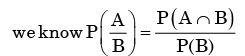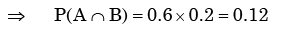QUESTION: 4

A fair coin is tossed 10 times. What is the probability that ONLY the first two tosses will yield heads?

Solution:

Let A be the event that first toss is head

And B be the event that second toss is head.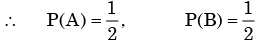By the given condition rest all 8 tosses should be tail

∴ The probability of getting head in first two cases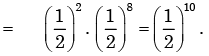QUESTION: 5

Consider the continuous random variable with probability density function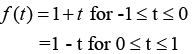The standard deviation of the random variables is

Solution: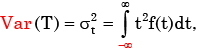T being the random variable of f(t).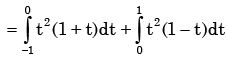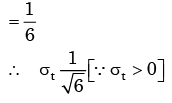QUESTION: 6

The probability that two friends share the same birth-month is

Solution:

Let A = the event that the birth month of first friend

And B= that of second friend.

∴ P( A )= 1, as 1st friend can born in any month

and P(B) = 1/12, by the condition.

∴ Probability of two friends share same birth-month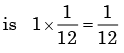QUESTION: 7

A box contains 5 block balls and 3 red balls. A total of three balls are picked from the box one after another, without replacing them back. The probability of getting two black balls and one red ball is

Solution:

Here the possible combination of picking up three balls without replacement is  BBR,  BRB,  RBB.

(B = Black ball,  R = Red balls)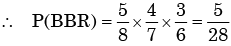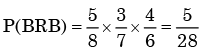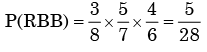∴ Probability of getting two black balls and one red ball is 5/28.

QUESTION: 8

Two dice are thrown. What is the probability that is the sum of the numbers on the two dice is eight?

Solution:

Here sample space = 6 × 6 = 36

Here, there are five such points whose sum is 8. They are (2,6), (3,5), (4,4), (5,3), (6,2).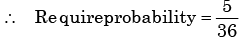QUESTION: 9

Arrivals at a telephone booth are considered to be poison, with an average time of 10 minutes between successive arrivals. The length of a phone call is distributes exponentially with mean 3 minutes. The probability that an arrival does not have to wait before service is

Solution: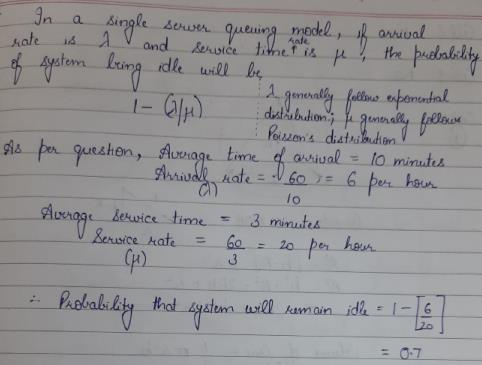QUESTION: 10

A box contains 5 black and 5 red balls. Two balls are randomly picked one after another from the box, without replacement. The probability for both balls being red is

Solution:

The probability of drawing two red balls without replacement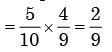QUESTION: 11

A lot has 10% defective items. Ten items are chosen randomly from this lot. The probability that exactly 2 of the chosen items are defective is

Solution:

Let A be the event that items are defective and B be the event that items are non- defective

∴ P( A )= 0.1 and P(B) = 0.9

∴ Probability that exactly two of those items are defective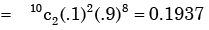QUESTION: 12

A box contains 20 defective items and 80 non-defective items. If two items are selected at random without replacement, what will be the probability that both items are defective?

Solution:

The probability of defective  items = 20/100

Therefore the probability of first two defective items without replacement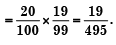QUESTION: 13

If three coins are tossed simultaneously, the probability of getting at least one head is

Solution:

Here the sample space S =23= 8.

No. of ways to get all tails =1.

∴ probability to get all tails = 1/8

∴ Probability to get at least one head is = 1-1/8= 7/8

QUESTION: 14

If 20 per cent managers are technocrats, the probability that a random committee of 5 managers consists of exactly 2 technocrats is

Solution:

The probability of technocrats manager = 20/100 = 1/5

∴ Probability of non technocrats manager = 4/5

Now the require probability  ​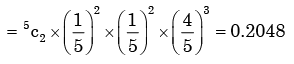QUESTION: 15

Four arbitrary point (x1,y1), (x2,y2), (x3,y3), (x4,y4), are given in the x, y – plane Using the method of least squares, if, regressing y upon x gives the fitted line y = ax + b; and regressing y upon x given the fitted line y = ax + b; and regressing x upon y gives the fitted line x = cy + d then

Solution:

y =ax+b − (i) and x = cy + d − (ii)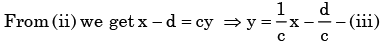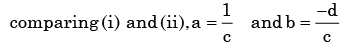QUESTION: 16

Chebyshev’s inequality states that the probability of a “Six Sigma” event is less than :

Solution:

If a bell curve is assumed, the probability of a “six sigma” event is on the order of one ten millionth of a percent.

QUESTION: 17

Three values of x and y are to be fitted in a straight line in the form y = a + bx by the method of least squares. GivenΣx = 6, Σy = 21, Σx2 = 14 and Σxy = 46, the values of a and b are respectively.

Solution: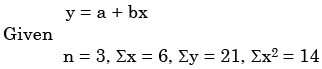​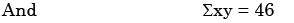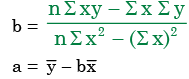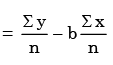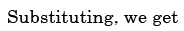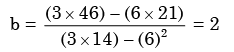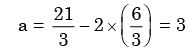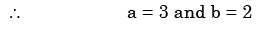QUESTION: 18

A box contains 10 screws, 3 of which are defective. Two screws are drawn at random with replacement. The probability that none of the two screws is defective will be

Solution:

A = First drawn screw non-defective.

B = Second drawn screw non-defective.

P(A) = 7/10

Because 7 out of 10 screws are non-defective and we sample at random,

P(B) = 7/10

The second drawing is the same as the beginning.

P(A Intersection B) = P(A) * P(B) = 0.7 * 0.7 = 0.49

It is Converted to percentage = 0.49 * 100 = 49%

The probability of two screws are defective is 49%.

QUESTION: 19

Which one of the following statements is NOT true?

Solution:
QUESTION: 20

If the standard deviation of the spot speed of vehicles in a highway is 8.8 kmph and the mean speed of the vehicles is 33 kmph, the coefficient of variation in speed is

Solution: